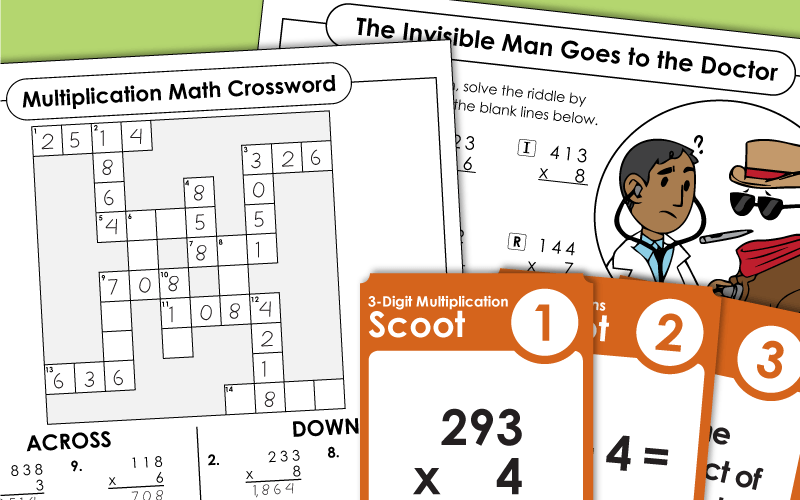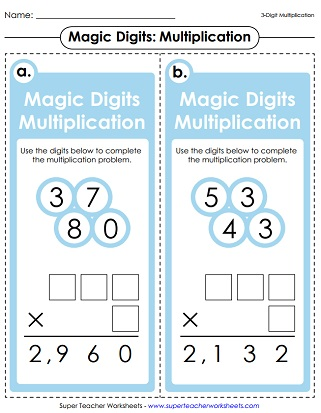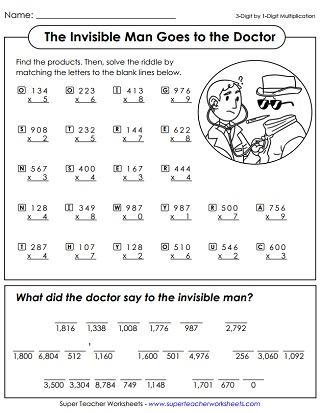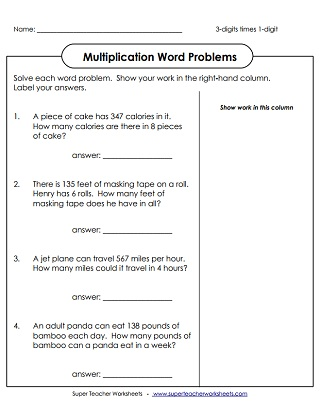# Multiplication Worksheets (3-Digits by 1-Digit)

This printable worksheets and activities on this page feature 3-digit times 1-digit multiplication problems. Download task cards, a Scoot! game, word problem practice pages, and math riddle worksheets. Most files are aligned with the Common Core standards.## 3-Digit Times 1-DigitPrintables

Solve these 3-digit numbers by 1-digit problems. Includes 10 vertical problems and 2 word problems.
What did the doctor say to the invisible man? To find the funny answer to this riddle, students will solve a set of 3-digit by 1-digit multiplication problems.
This activity has shapes with numbers written in them. Students multiply numbers in similar shapes together. For example: Find the product of the numbers in the octagons.
Multiply to find each product.  The answers are used to complete the math crossword puzzle.
Find the answer to each multiplication problems, then color the answers on the bingo boards.
This worksheet has 3-digit by 1-digit horizontal multiplication problems. Students rewrite each problem vertically and solve. (example: 772 x 9)
Examine the solved math problems. There are errors in the solutions. Explain the errors and then re-solve.
Your kids will enjoy this secret code multiplication activity. First they'll use the cipher key to decode the picture symbols into numbers. Then they will multiply to find the products.
Color the picture of the giraffe and multiply to find the products. All problems include 3-digit by 1-digit numbers.
Graph Paper Math Drills; 3-digits times 1-digit (example: 523 x 8)
Solve the multiplication problems and word problems (example: 446 x 8)
This worksheet includes a variety of 3-digit by 1-digit multiplication problems, including word problems and an input/output table.
Multiplication facts with sea animal pictures; 3-digits times 1-digit (example: 224 x 6)
A selection of three-digit by one-digit multiplication word problems. (example: 456 x 9)
Multiply basic 2-digit by 1-digit, 3-digit by 1-digit, and multiplying money by 1-digit factors. (example: 421 x 6)
Use the grid to decode and solve the problems. (example: 909 x 7)
Multiply 3-digit by 1-digit numbers, complete the in and out box, and fill in the multiplication arrow chain (example: 223 x 3)
More practice with multiplying 2-digit by 1-digit and 3-digit by 1-digit. (example: 657 x 8)

Multiplication Worksheet Generator

Create your own multiplication worksheets! You choose the number of digits in the factors. Also tell if you would like to include money or word problems.

When students play this game, they will solve 3-digit by 1-digit multiplication problems on task cards. A task card is placed on each student desk. Each time the teacher says Scoot!, students move to a different desk, and solve another math problem.
Use the digits to complete the multiplication problem. Includes 12 task cards. This activity can be used as a learning center, or game for small groups of students.
Here's a set of 30 task cards that you can use for classroom games, study sessions, or learning centers.

## Multiplication Patterns

Place value patterns in multiplication; Learn to use mental math to multiply large numbers that end in zero (example: 400 x 6)
More Multi-Digit Multiplication

Browse our incredible selection of multi-digit multiplication worksheets.

## Sample Worksheet ImagesMy Account
Site Information